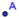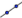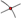# Meeting on the Dot

## OBJECTIVE: To learn how to find the intersection of two lines graphically

In a previous lesson, you discovered two special pairs of lines: parallel lines and perpendicular lines. Watching 'Em Dance: https://www.geogebra.org/m/arfhcasa In another lesson, you learned a more general way of classifying pairs of lines: inconsistent (parallel lines), consistent and independent (intersecting lines), or dependent (coincident lines or same line). Working in Pairs: https://www.geogebra.org/m/hvtw2rfc Using the applet below, you will be able to explore intersecting lines in greater detail. There is a postulate in Geometry that states that when two lines intersect, they intersect at a point. In this lesson, you will be asked to find the point of intersection of pairs of lines. Using thePoint Tool and/or theLine Tool, graph each pair of lines listed below. Remember to convert the lines to either the STANDARD FORM (Ax + By = C) or the SLOPE-INTERCEPT FORM (y = mx + b) and use the corresponding graphing technique in each case. Then use theIntersect Tool to find the point of intersection of each pair of lines. Record your answers in the Question Box that follows. Pair 1 : 5x + 4y = 20 & 2x - y = -18 Pair 2 : x = 5y - 10 & 4x + 28 = 14y Pair 3 : 4x = 2y - 2 & 3y = 21 - 3x

QUESTION: What is the point of intersection of Pair 1? Pair 2? Pair 3?

QUESTION: In performing the tasks here, did you keep both the Algebra View and Geometry View open? Do you think it would have been better if you kept just one view open? Briefly explain.

## Keep on practicing using the applet below.

﻿ In the applet below, note the system of equations displayed. One equation is displayed in pink. The other equation is displayed in purple. Directions: 1) Move the pink points (of the pink line) so that this pink line becomes the graph of the pink equation displayed. Note that the equations are written in SLOPE-INTERCEPT FORM. 2) Move the purple points (of the purple line) so that this purple line becomes the graph of the purple equation displayed. 3) Enter the respective x- and y- coordinates of the solution to this system of equations. If you enter this correctly, the applet will confirm this. Repeat as many times as needed to master the concept.

## TODAY you learned how to find the point of intersection of two lines graphically.

In future lessons, you'll learn how to find the points of intersection algebraically. Hope you ENJOYED today's lesson!